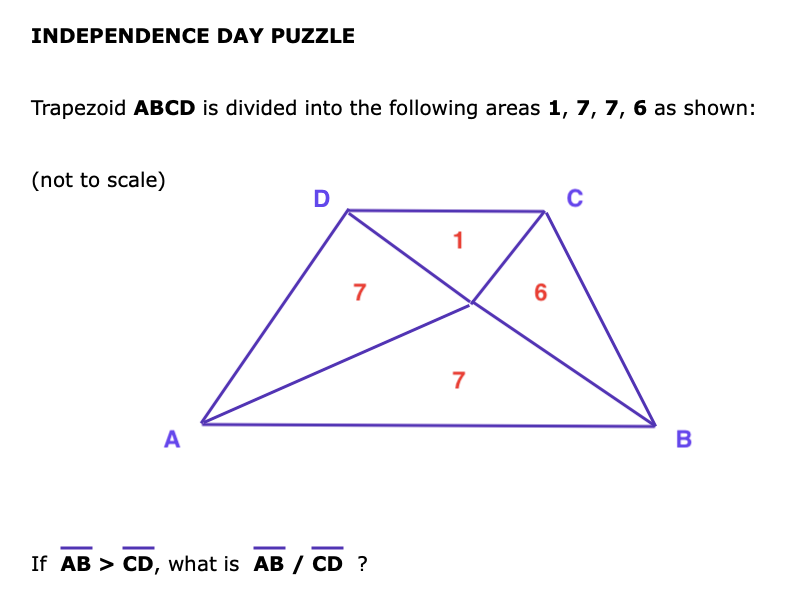# INDEPENDENCE DAY PUZZLE - JULY 4, 2021$$\frac{13 + \sqrt{141}}{2}$$
SOLUTION:
$$\text{Let}\ AB = r \text{ and}\ CD = s$$
Also let:
$$X \text{ be height of triangle with base}\ AB$$
$$Y \text{ be be height of triangle with base}\ CD.$$
Using formula for each triangle, the total height of trapezoid is
$X+Y = \frac {14}{r} + \frac 2s$
Now use formula for area of trapezoid:
$21 = \frac12\cdot (X+Y)\cdot (AB+CD) = \frac12\left(\frac {14}{r} + \frac 2s\right)(r+s) = 8 + 7\cdot\frac sr + \frac rs$
Or
$\frac rs + 7\cdot\frac sr - 13 = 0$
Now let $$\rho = \frac rs$$ then $$\rho + 7\rho^{-1} - 13 = 0$$
Or $$\rho^{2} -13 \rho +7 = 0$$ Quadratic formula yields: $$\rho = \frac{13\pm\sqrt{141}}{2}$$
Since $$\rho = \frac rs = AB/CD > 1$$ answer is: $$\frac{13 + \sqrt{141}}{2}$$